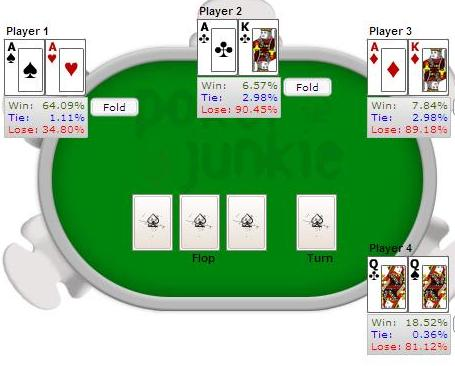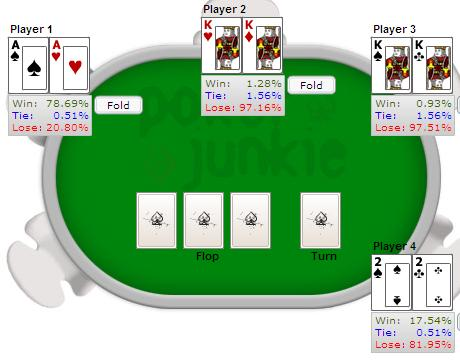> > > Folding Aces Pre Flop - Example Calculation

# Folding Aces Pre Flop - Example Calculation

27 May 2008, By: Pokerjunkie.comimg 2599

As you can see in the pictures below (from the poker odds calculator), it's more or less impossible for you to have less than 50% equity in the pot if you call.

Let's make some calculations to see where we stand. First we need to simplify things a bit.

### Assumptions

Let's make a couple of assumptions for the calculation:

• If you call, your winning chances are 50%.
• The pot will not be split (see pictures below).
• With one opponent left, your chances to win first prize equals your share of the total chips.

The last point is usually thought to hold true if you're as good a player as your opponent.

### Calculation

Now, you can either fold or call, and then the following things can happen:

#### Fold

• Second place secured
• 20,000 in chips
• One opponent with 240,000
• 8% chance at first place

#### Call and win (50%)

• Second place secured
• 80,000 in chips
• One opponent with 180,000
• 31% chance at first place

#### Call and lose (50%)

• Fourth place
• Zero dollars and no chances

Your expectation for folding or calling can be written like this:

E(fold) = S + 0.08*(F-S)
E(call) = 0.5*S + 0.31*(F-S)

where S is second prize and F is first prize.

According to this, the expectation is larger for folding than for calling only if the following is true:

S > 0.85 F

Usually second prize is not this big; it's usually around half the size of the first prize.

However, in a satellite S usually equals F, In this case a fold is an easy choice.

### Conclusion

With these assumptions, calling beats folding even though you risk being eliminated on fourth place and win zero.

Again, this assumes that the prize money is not extremely important to you. If it is, folding certainly makes sense.

### Some possible match-ups

Results from the poker odds calculator:## Latest News

 1BetOnline \$2,500